# QCD

Although LEP was principally designed to perform precision tests of the electroweak theory and the Z0, it also provides an excellent environment for testing the strong interaction sector of the standard model, QCD (Quantum ChromoDynamics). In this work, the Cambridge OPAL group has often collaborated with Bryan Webber and the Cavendish HEP Theory Group.

Approximately 70% of the time, the Z boson decays to quarks like this: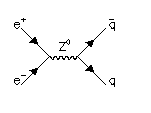The quarks then form jets of hadrons like this. The strong interaction between quarks is mediated by "gluons", and sometimes one of the quarks may emit an energetic gluon like this: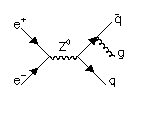In this case an event containing three jets of hadrons may be seen. By studying such events we can test QCD.

The fundamental parameter governing QCD is the quark-gluon coupling constant alpha_s. It is therefore important to measure this fundamental constant of nature as accurately as we can. One can measure alpha_s most simply by counting the numbers of jets in events, but many other techniques are also interesting. At Cambridge we have studied a number of methods of measuring alpha_s, using a new type of QCD calculation developed by our Cavendish colleagues, based on resummation. The result is alpha_s=0.120+-0.006, where the error is dominated by uncertainties surrounding the theoretical calculations. If you click here you can see a summary of the results. Seven separate measurements were made, which are all compatible with the mean value (shown by the band). This consistency is a valuable test of the theory. You can read the whole paper by clicking here.

When higher orders in QCD are considered, more complicated processes can take place in which, for example, a gluon may split into quark-antiquark, or into two gluons.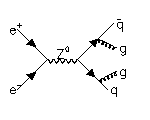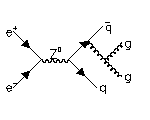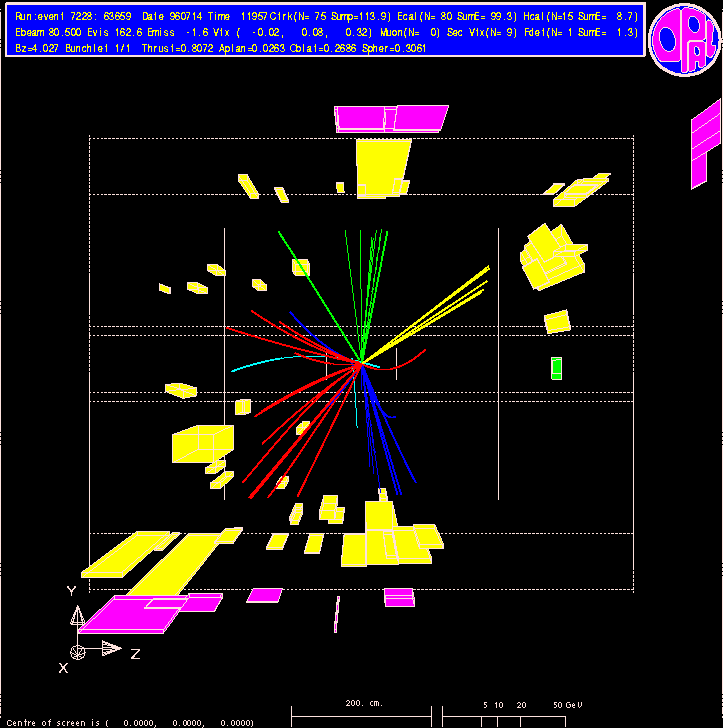The relative probabilities of these happening are fully predicted by QCD, and depend on the nature of the underlying symmetry group (which is SU(3) in standard QCD). We have shown that distributions of observables which measure the shape of events are sensitive to these group constants, CF (governing the g->qg splitting), CA (the number of colours which determines the g->gg splitting), and TR (which defines the g->qq vertex). If you click here you can see the measured values of pairs of these colour factors. The data are found to be consistent with standard QCD.

Algorithms for identifying jets of hadrons are extensively used, because the jets reflect the underlying quark-gluon structure of the event. Traditionally, quite different algorithms have been applied in e+e- experiments like OPAL, and in hadron-hadron colliders. Recently the Cambridge group has introduced a jet-finder for e+e- collisions which is essentially identical to that used at the Fermilab pbar-p collider. The idea is to classify particles lying within a cone of some fixed angle R as a jet, where the axis of the cone coincides with the momentum sum of the particles contained. It is then possible to compare jets created in Z0 decays with those formed in pbar-p collisions, as shown here. The figure shows the energy flow within smaller cones of radius r contained within the jet. We find that the jets in Z decays are significantly narrower, and the probable explanation is that the jets in Z0 decays are mainly quark jets, while those formed in the CDF pbar-p experiment are principally gluons. The gluon has a larger colour charge than a quark, and thus fragments into a broader jet. You can read the whole paper by clicking here.